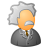# New to Qlik Sense

If you’re new to Qlik Sense, start with this Discussion Board and get up-to-speed quickly.

Announcements
QlikWorld 2022, LIVE in Denver CO., May 16-19, 2022. REGISTER NOW TO RECEIVE EARLY BIRD PRICING
cancel
Showing results for
Did you mean:Contributor III

## mode of quantity

Hello,

I have a table where I count The sum of quantity using variables ( min and max quantity, min and max visit)

I use this formula

Sum(

aggr(

If(

((Sum(Quantity) >= \$(MinVisit) and Sum(Transaction) < \$(MaxVisit)) and (Sum(Quantity) >= \$(MinQuantity) and Sum(Quantity) < \$(MaxQuantity)))

,  Quantity )

,SalesID)

)

I want to calculate the mode of quantity--- mode(Quantity)  but when I use the variables it should change appropriately

4 RepliesMVP

Are you checking both MinVisit variable against Sum(Quantity) or should that be Sum(Transaction)??

Sum(

aggr(

If(

((Sum(Quantity) >= \$(MinVisit) and Sum(Transaction) < \$(MaxVisit)) and (Sum(Quantity) >= \$(MinQuantity) and Sum(Quantity) < \$(MaxQuantity)))

,  Quantity )

,SalesID)

)

what exactly do you mean by this? Can you elaborate with an example?

"I want to calculate the mode of quantity--- mode(Quantity)  but when I use the variables it should change appropriately"Contributor III
Author

This is Formula where I Calculate Sum Quantity and now I want to calculate Mode of this Quantity. Using same variables Than I used calculating sum of Quantities.and How can I calculate mode of Quantity restricted with this variables?MVP

I am not sure I understand... would you be able to share some sample data and explain the output you are looking to get?MVP

Hi, I'm not sure to understand, if you change Sum to Mode it doesn't works?

Mode(

aggr(

If(

((Sum(Quantity) >= \$(MinVisit) and Sum(Transaction) < \$(MaxVisit)) and (Sum(Quantity) >= \$(MinQuantity) and Sum(Quantity) < \$(MaxQuantity)))

,  Quantity )

,SalesID)

)Tags
Community Browser